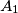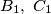### IMO Shortlist 2001 problem G1

Kvaliteta:
Avg: 0.0
Težina:
Avg: 6.0
Let$A_1$ be the center of the square inscribed in acute triangle$ABC$ with two vertices of the square on side$BC$. Thus one of the two remaining vertices of the square is on side$AB$ and the other is on$AC$. Points$B_1,\ C_1$ are defined in a similar way for inscribed squares with two vertices on sides$AC$ and$AB$, respectively. Prove that lines$AA_1,\ BB_1,\ CC_1$ are concurrent.
Source: Međunarodna matematička olimpijada, shortlist 2001